# NCERT Solutions for Class 10 Maths Chapter 13 Surface Areas and Volumes Ex 13.2

Unless stated otherwise, take π = 227

Question 1.
A solid is in the shape of a cone standing on a hemisphere with both their radii being equal to 1 cm and the height of the cone is equal to its radius. Find the volume of the solid in terms of n.
Solution:
Radius of the hemisphere = 1 cm
Volume of the hemisphere = 23πr³ = 23π(1)³ = 23πcm³
Radius of base of the cone = 1 cm
Height of the cone = 1 cmVolume of the cone = 13πr²h = 13πr² x 1 = 13π cm³
Total volume of the solid = Volume of the hemisphere + Volume of the cone
23π cm³ + 13π cm³ = π cm³

Question 2.
Rachel, an engineering student, was asked to make a model shaped like a cylinder with two cones attached at its two ends by using a thin aluminium sheet. The diameter of the model is 3 cm and its length is 12 cm. If each cone has a height of 2 cm, find the volume of air contained in the model that Rachel made. (Assume the outer and inner dimensions of the model to be nearly the same.)
Solution:
Volume of air contained in the model = Total volume of the solid
Diameter of base of each cone = 3 cm
∴ Radius of base of each cone = 32
Height of each cone = 2 cmQuestion 3.
A gulab jamun, contains sugar syrup up to about 30% of its volume. Find approximately how much syrup would be found in 45 gulab jamuns, each shaped like a cylinder with two hemispherical ends with length 5 cm and diameter 2.8 cm (see figure).Solution:
Volume of one piece of gulab jamun
= Volume of the cylindrical portion + Volume of the two hemispherical ends 1 2 8
Radius of each hemispherical portion = 2.82 = 1.4 cmVolume of both hemispherical ends = 2 x 5.74 cm³ = 11.48 cm³
Height of the cylindrical portion = (total height) – (radius of both hemispherical ends)
= 5 cm – 2(1.4) cm = 5 cm – 2.8 cm = 2.2 cm
Radius of the cylindrical portion = 1.4 cm
Volume of the cylindrical portion of gulab jamun = πr²h
227 x (1.4)² x 2.2cm³
22×1.4×1.4×2.27cm³ = 13.55 cm³
Total volume of one gulab jamun = Volume of the two hemispherical ends + Volume of the cylindrical portion
= 11.48 cm³ + 13.55 cm³ = 25.03 cm³
Volume of sugar syrup = 30% of volume of gulab jamun
30100 x 25.03 cm³ = 7.50 cm³
∴ Volume of sugar syrup in 45 gulab jamuns
= 45 (volume of sugar syrup in one gulab jamun)
= 45 x 7.50 cm³ = 337.5 cm³ = 338 cm³ approx.

Question 4.
A pen stand made of wood is in the shape of a cuboid with four conical depressions to hold pens. The dimensions of the cuboid are 15 cm by 10 cm by 3.5 cm. The radius of each of the depressions is 0.5 cm and the depth is 1.4 cm.
Find the volume of wood in the entire stand (see figure).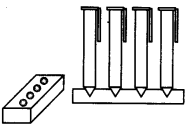Solution:
Radius of one conical depression = 0.5 cm
Depth of one conical depression = 1.4 cm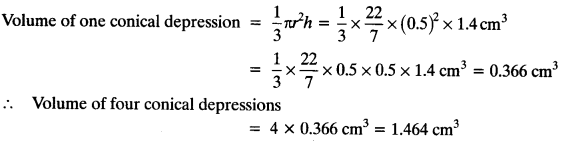Volume of cuboidal box = l x b x h
= 15 x 10 x 3.5 cm³
= 525 cm³
Remaining volume of box = Volume of cubical box – Volume of four conical depressions
= 525 cm³ – 1.464 cm³ = 523.5 cm³

Question 5.
A vessel is in the form of an inverted cone. Its height is 8 cm and the radius of its top, which is open, is 5 cm. It is filled with water up to the brim. When lead shots, each of which is a sphere of radius 0.5 cm are dropped into the vessel, one-fourth of the water flows out. Find the number of lead shots dropped in the vessel.
Solution: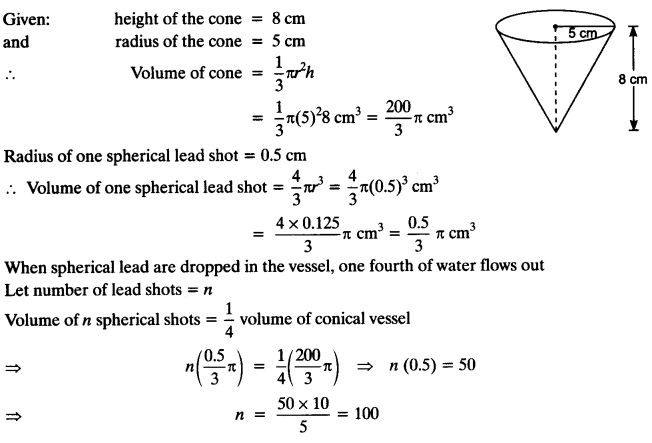Question 6.
A solid iron pole consists of a cylinder of height 220 cm and base diameter 24 cm, which is surmounted by another cylinder of height 60 cm and radius 8 cm. Find the mass of the pole, given that 1 cm3 of iron has approximately 8 g mass. (Use π = 3.14)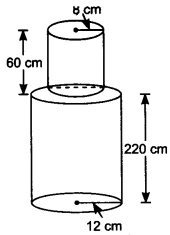Solution:
Given: radius of 1st cylinder = 12 cm
and height of 1st cylinder = 220 cm
∴ Volume of 1st cylinder = πr²h
= π(12)² (220) cm³
= 144 x 220π cm³
= 144 x 220 x 3.14 cm³
= 99475.2 cm³ … (i)
Given: radius of 2nd cylinder = 8 cm
and height of 2nd cylinder = 60 cm
∴ Volume of 2nd cylinder = πr²h
= π(8)² (60) cm³ = 64 x 60π cm³
= 64 x 60 x 3.14 cm³
= 12057.6 cm³ … (ii)
Total volume of solid = Volume of 1st cylinder + Volume of 2nd cylinder
= 99475.2 cm³ + 12057.6 cm³ = 111532.8 cm³
Given: mass of 1 cm³ of iron = 8 g
∴ Mass of 111532.8 cm³ of iron = 111532.8 x 8 g
= 892262.4 g = 892.262 kg

Question 7.
A solid consisting of a right circular cone of height 120 cm and radius 60 cm standing on a hemisphere of radius 60 cm is placed upright in a right circular cylinder full of water such that it touches the bottom. Find the volume of water left in the cylinder, if the radius of the cylinder is 60 cm and its height is 180 cm.
Solution:
Radius of hemisphere = 60 cm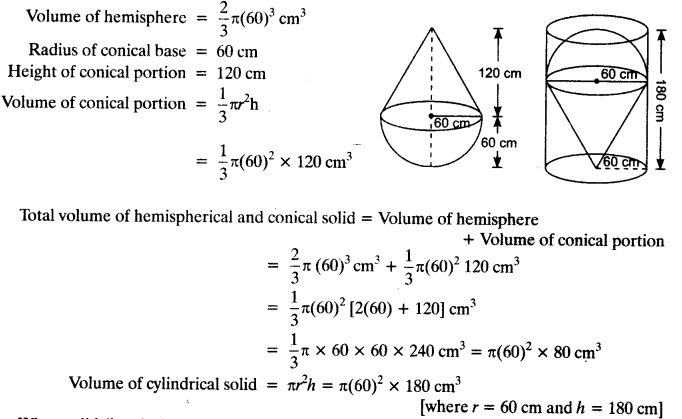When solid (hemisphere + conical) is kept in cylindrical solid, then volume of water left in cylinder
= Volume of cylinder – (Volume of hemisphere + Volume of cone)
= [π(60)² x 180 – π(60)² x 80] cm³
= π(60)² [180 – 80]cm³ = π x 3600 x 100 cm³ = 1130400 cm³ = 1.130 m³

Question 8.
A spherical glass vessel has a cylindrical neck 8 cm long, 2 cm in diameter, the diameter of the spherical part is 8.5 cm. By measuring the amount of water it holds, a child finds its volume to be 345 cm³. Check whether she is correct, taking the above as the inside measurements, and π = 3.14.
Solution:
Volume of water the glass vessel can hold = 345 cm³ (Measured by the child)
Radius of the cylindrical part = 22 = 1 cmHeight of the cylindrical part = 8 cm
∴ Volume of the cylindrical part = πr²h
= 3.14 x (1)² x 8 cm³
Diameter of the spherical part Radius = 8.5 cm
∴ Radius = 8.52 cm = 8520Total volume of the glass vessel = Volume of the cylindrical part + Volume of the spherical part
= 25.12 cm³ + 321.39 cm³ = 346.51 cm³
Volume measured by child is 345 cm³, which is not correct. Correct volume is 346.51 cm³.